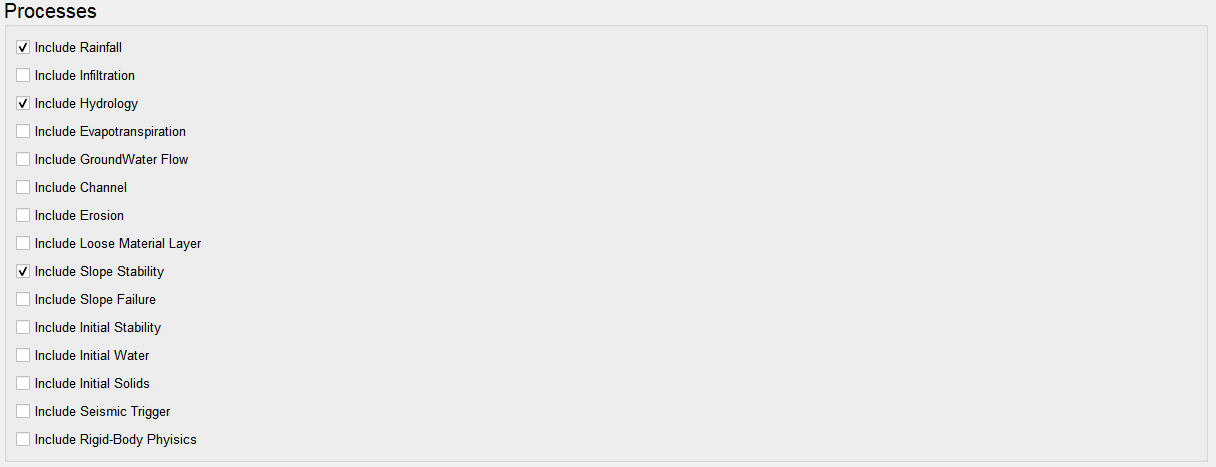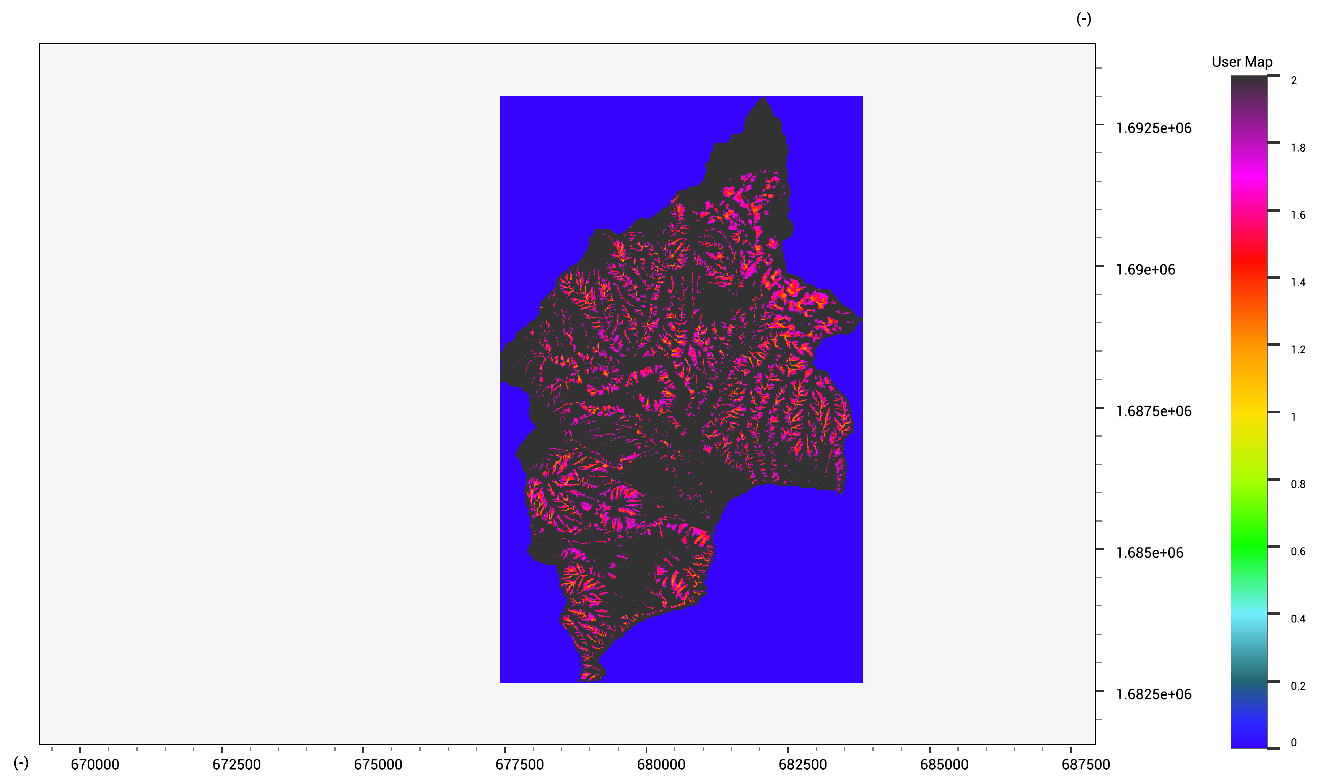LISEM Classic

LISEM

# SlopeFailure

### Slope failure volumes with LISEM

Simulation of slope stability and failure using LISEM require the setup of full hydrology. Afterwards, the relevant processes can be turned on.The required data is available for download for the Grande Bay Area: Cohesion, Internal Friction Angle and Soil Density. The modelling results now show the factor of safety (critical area can be highlighted by looking at values between 0.5 and 2.0.The initial stability option can be used to perform auto-calibration so that at the start of a simulation, all pixels are at least stable with a minimal margin. Any location that is sufficiently close to 1.0, will fail during an event due to increased pore pressures, water weight or other factors.

### Slope stability with the Infinite Slope

Simulation using the infinite slope model. The infinte slope model can also be run within the scripting environment. Using all the same maps as are used for the model:

FoS.map = (coh.map + (thetai.map * 1000.0 + thetas.map * soildensity.map)* tan(ifa.map) * sin(atan(slope(dem.map))) * cos(atan(slope(dem.map))))/((thetai.map * 1000.0 - thetas.map * soildensity.map)* cos(atan(slope(dem.map)))* cos(atan(slope(dem.map))));